## Thursday, 7 August 2014

### chapter-14 Worked Out Examples

 Example: 11
 For two events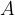$A$ and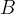$B$, find the probability that exactly one of the two events occur.
 Solution: 11
This can happen in two ways:
 Way Set of Outcomes$A$ occurs,$B$ doesn’t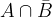$A \cap \bar B$$B$ occurs,$A$ doesn’t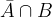$\bar A \cap B$
Note that the two ways are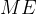$ME$. Thus, the required probability is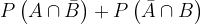$P\left( {A \cap \bar B} \right) + P\left( {\bar A \cap B} \right)$, which from the second relation becomes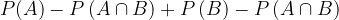$P(A) - P\left( {A \cap B} \right) + P\left( B \right) - P\left( {A \cap B} \right)$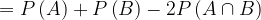$= P\left( A \right) + P\left( B \right) - 2P\left( {A \cap B} \right)$
Justify this last expression using a Venn diagram, by shading the area it represents.
 Example: 12
For two events$A$ and$B$, show that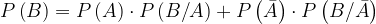$P\left( B \right) = P\left( A \right) \cdot P\left( {B/A} \right) + P\left( {\bar A} \right) \cdot P\left( {B/\bar A} \right)$
 Solution: 12
This is straightforward, since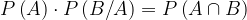$P\left( A \right) \cdot P\left( {B/A} \right) = P\left( {A \cap B} \right)$ recall the discussion in the previous section and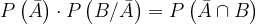$P\left( {\bar A} \right) \cdot P\left( {B/\bar A} \right) = P\left( {\bar A \cap B} \right)$
Now, since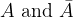$A\,\,{\rm{and}}\,\,\bar A$ are mutually exclusive, we must have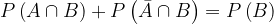$P\left( {A \cap B} \right) + P\left( {\bar A \cap B} \right) = P\left( B \right)$